# Lecture 16 Stability and Matrix Norms¶

In this lecture we introduce matrix norms as a way to understand the amount in which a matrix blows up an error. Consider an approximation of $A \mathbf x$ given by

$$A(\mathbf x + \Delta \mathbf x)$$

where $A$ is $n \times m$, $\mathbf x$ is $m \times 1$ and $\Delta \mathbf x$ is $m \times 1$ representing a small perturbation of $\mathbf x$. This is, assume for a suitable norm that

$$|{\Delta \mathbf x}\|\leq \epsilon$$

The error of this approximation is precisely

$$A(\mathbf x + \Delta \mathbf x)-A\mathbf x = A(\Delta \mathbf x)$$

We want a way to measure $\|{A (\Delta \mathbf x)}\|$. This will be accomplished by defining an induced matrix norm.

## Induced matrix norms¶

Suppose $A$ is an $n \times m$ matrix, and consider two norms $\| \mathbf v\|_X$ for $\mathbf vd\mathbb R^m$ and $\| \diamond\|_Y$ on $\mathbb R^n$. For example, they could both be the 2-norm, both be the $\infty$-norm, or a mixture of the two. These two norms induce a norm on matrices:

$$\|A\|_{X \rightarrow Y} \triangleq \sup_{\mathbf v : \|\mathbf{v}\|_X=1} \|A \mathbf v\|_Y$$

That is, take the supremum of $\|{A \mathbf v}\|_Y$ over the set of all vectors $\mathbf v$ whose X-norm is one.

When we use the same vector norm for the domain and range, we specify only one space

$$\|A\|_X \triangleq \|A\|_{X \rightarrow X}$$

For the induced 2, 1, and $\infty$-norm we use

$$\|A\|_2, \|A\|_1 \qquad \hbox{and} \qquad \|A\|_{\infty}.$$

## Induced matrix norms are norms¶

In lectures we saw that it is easy to prove that induced norms are norms: they satify three properties

1. $\|c A \| = |c| \|A\|$
2. $\| A + B \| \leq \|A\| + \|B\|$
3. $\|A\| =0 \Rightarrow A = 0$

The last property was shown using an equivalent definition of the induced norm:

$$\|A\| = \sup_{\mathbf v} {\|A \mathbf v\| \over \| \mathbf v\|}$$

This follows since we can scale $\mathbf v$ by its norm so that it has unit norm, that is, ${\mathbf v} \over \|\mathbf v\|$ has unit norm. Then

$$\sup_{\mathbf v} {\|A \mathbf v\| \over \| \mathbf v\|} = \sup_{\mathbf v} {\|A {\mathbf v \over \mathbf \|\mathbf v\|}\|} \leq \sup_{\mathbf v : \|\mathbf w \| = 1} {\|A w \|} = \|A\|$$

This combined with the trivial case

$$\sup_{\mathbf v} {\|A \mathbf v\| \over \| \mathbf v\|} \geq \sup_{\mathbf v : \|\mathbf v \| = 1 } {\|A \mathbf v\| \over \| \mathbf v\|} = \sup_{\mathbf v : \|\mathbf v \| = 1 } {\|A \mathbf v\| } = \|A\|$$

## Extra properties of induced norms¶

We also have the following two additional proper

## Induced 2-norm¶

Induced norms define the "length" of a matrix by how much they magnify vectors. We can visualize this in 2D for the induced 2-norm $\|A\|_2$, taking a random 2 x 2 matrix.

In :
using PyPlot

A=rand(2,2)

Out:
2x2 Array{Float64,2}:
0.692806  0.330373
0.809566  0.617713

The set $\{\mathbf v : \|\mathbf v\|_2 =1\}$ is the unit circle:

In :
t=linspace(0.,2π,100)
x=cos(t)
y=sin(t)

plot(x,y);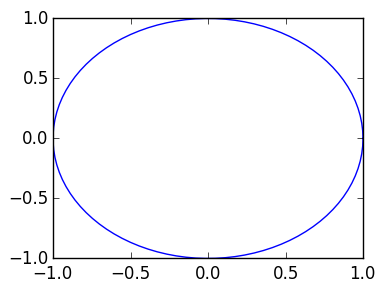For each $\mathbf v$ in the circle, we see where $A\mathbf v$ is mapped to:

In :
Ax=copy(x)
Ay=copy(y)

for k=1:length(x)
Ax[k],Ay[k]=A*[x[k],y[k]]
end
plot(Ax,Ay);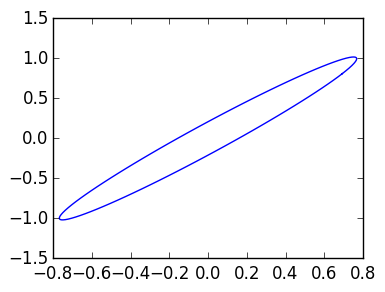Out:
1-element Array{Any,1}:
PyObject <matplotlib.lines.Line2D object at 0x30b8eebd0>

The induced norm of $A$ is then the maximum value of $\|A\mathbf v\|_2$. This is calculated using the inbuilt norm function:

In :
norm(A)

Out:
1.268895038744742

## Induced $\infty$-norm¶

We can do the same experiment to visualize the $\infty$-norm. Here we use the notation [v;w;z] where v, w and z are Vectors to concatenate:

In :
v=[1,2,3]
w=[5,6,7]
z=[9,8,5]

[v;w;z]

Out:
9-element Array{Int64,1}:
1
2
3
5
6
7
9
8
5

We begin by plotting the set $\{\mathbf v : \|\mathbf v\|_\infty =1\}$, which is the unit square.

In :
x=[linspace(-1.,1.,100);  ones(100);
linspace(1.,-1,100);
-ones(100)
]  #; concatonates vectors
y=[-ones(100);            linspace(-1.,1.,100);
ones(100);
linspace(1.,-1.,100)
]

plot(x,y);

axis([-2,2,-2,2]);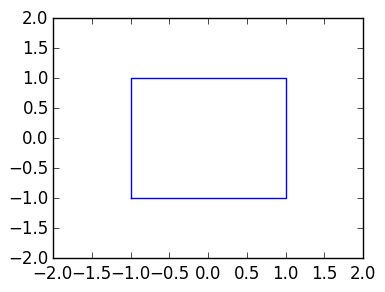We multiply this new set of vectors by $A$ and plot the result.

In :
Ax=copy(x)
Ay=copy(y)

for k=1:length(x)
Ax[k],Ay[k]=A*[x[k],y[k]]
end
plot(Ax,Ay);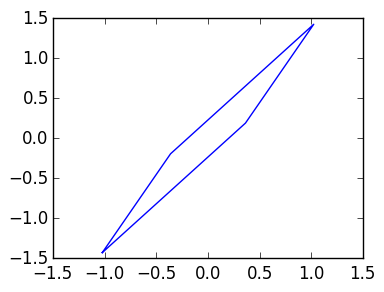To calculate $\|A\|_\infty$, we need to measure the maximum value in the $\infty$ norm. The built-in function norm(A,Inf) does this.

In :
norm(A,Inf)

Out:
1.4272791819737858

We can visualize this by drawing the unit square scaled by this norm, and showing that it passes through the largest point:

In :
nrm∞=norm(A,Inf)
plot(Ax,Ay)
plot(nrm∞*x,nrm∞*y)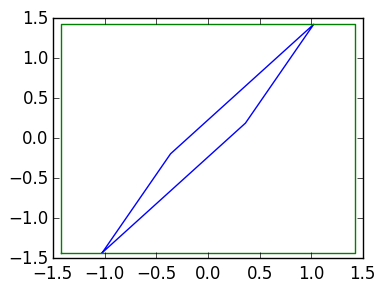Out:
1-element Array{Any,1}:
PyObject <matplotlib.lines.Line2D object at 0x30bd8ffd0>

# Induced $\infty$ to 2 norm¶

We can also use two different norms, for example,

$$\|A\|_{\infty \rightarrow 2}$$

measures how large the unit square is mapped to, measuring in the 2-norm.

There is no inbuilt command for calculating matrix norms when the norms differ. However, we can approximate it by finding the maximum of all vectors we used to plot the unit square:

In :
nrm∞to2=0.0
for k=1:length(x)
Av=[Ax[k],Ay[k]]
nrm∞to2 = max(nrm∞to2, norm(Av))
end

nrm∞to2

Out:
1.7561382947071715

This matches:

In :
plot(Ax,Ay)
plot(nrm∞to2*cos(t),nrm∞to2*sin(t))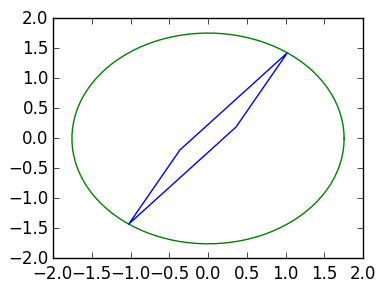Out:
1-element Array{Any,1}:
PyObject <matplotlib.lines.Line2D object at 0x30b0a0490>

## Norms measure how much a vector is magnified¶

$$|{\Delta \mathbf x}\|\leq \epsilon$$

The error of this approximation is precisely

$$A(\mathbf x + \Delta \mathbf x)-A\mathbf x = A(\Delta \mathbf x)$$
In :
x=rand(2)
ε=0.0001
Δx=ε*rand(2)
x̄=x+Δx       # x̄ is x perturbed by Δx
norm(x-x̄)

Out:
7.286987817147542e-5

Multiplication by $A$ magnifies the error by at most $\|A\|$:

In :
A=rand(2,2)
norm(A*x-A*x̄)   ≤    norm(x-x̄)*norm(A)

Out:
true

This holds true even if norm of $A$ is large:

In :
A=[1. 1000.; 0. 1.0]
norm(A)

Out:
1000.0009999990001
In :
norm(A*x-A*x̄) ≤ ε*norm(A)

Out:
true